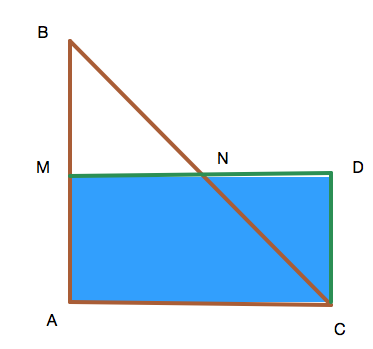# [G] Question 1: Easy Geometry

Geometry Level 1ABC is a right angle triangle with angle $BAC = 90^\circ$.
Let M and N be the midpoints of BA and BC respectively.
Let D be a point on MN extended such that CD//AB
The ratio of the area of quadrilateral AMDC to the area of triangle ABC is $\frac { a }{ b }$ where a and b are coprime integers.
Find a+b.

×